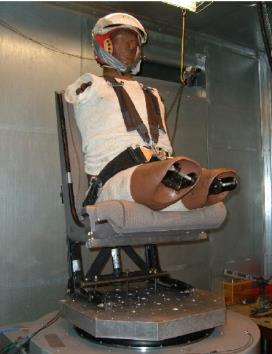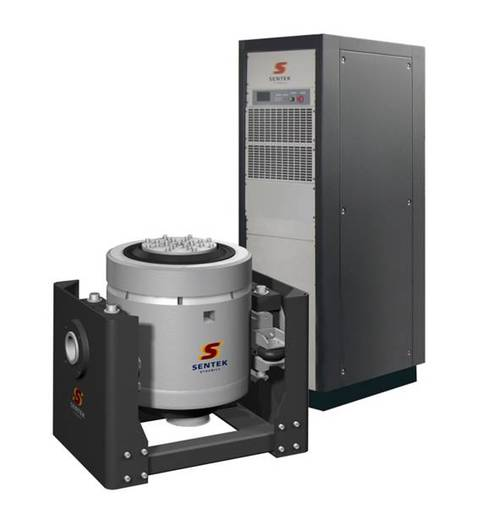# Need To apply external vibration

I would like to apply an external vibration with fixed frequency and amplitude on human body model in ANSYS. How can I do that?

«13

• What does you question have to do with ANSYS simulation software?

To shake an object, you can use a permanent magnet shaker, a power supply and a controller or signal generator.

If you are at a University in the USA, you need to have approval from an Institutional Review Board (IRB) before any human testing begins.

• edited April 2018

Actually, I want to simulate Whole body vibration (WBV) process in ANSYS. For that, I have to apply fixed frequency and fixed amplitude vibration from the bottom of seated/standing human model. So I want to how can I apply such kind of external vibration?

• It's the same thing on a bigger scale.  There are shaker tables that you can stand on or bolt a seat to and shake on a vertical axis.

The type of shaker table actuator depends on the frequency you want to apply.  If you want to go down into the single digit number of Hz, between 1 and 10 Hz, then you must use a hydraulic actuator. Lansmont is one company that makes hydraulic shaker tables. If your lower limit is above 10 Hz, then you can also use electro-dynamic shakers such as ones from Sentek.

Here is an image of a seat bolted to the armature of a shaker with a manequin strapped to the seat. The armature is driven by electromagnetic coils that are in the drum and powered by the power supply in the cabinet.• edited April 2018

Thanks for your reply. I want to simulate the whole process in ANSYS. How can I apply the shaker vibration in ANSYS? Can I do it by using ANSYS static structure module?

• You could use a Transient Structural system to simulate a shaker table. You could also use Harmonic Response since you are applying a sinusoidal input.

• I tried with Harmonic Response where I could apply force/acceleration as constant/tabular format. However, I could not apply acceleration/loads as function of time. How can I do that in Harmonic response module? What is the difference in applying load in static structural module and harmonic response module?

thanks.

• In Harmonic Response, when you apply a Load such as a force or acceleration, you are specifying the amplitude of the vibration.  It is assumed that the load is sinusoidal with time. During the solution, the frequency of the sinusoidal vibration is stepped from the minimum to the maximum frequency specified in the Analysis Settings. In Static Structural, the load is a fixed static value.

• Thanks for your reply. For example, If I want to apply a sinusoidal load in a particular direction having frequency 30 Hz, amplitude of .4 mm, then what should be the magnitude of force in the harmonic response module? For static structural module, what if I use this formula F=ma for having the solution? Does that mean that I will get the solution for a particular frequency only?

• Apply a displacement of 0.4 mm in the desired direction. In the Analysis Settings, you can specify 30 Hz for this Harmonic Response.

The maximum acceleration of a point vibrating at 30 Hz with an amplitude of 0.4 mm is 14.2 m/s^2 or 1.45 G. You could use this acceleration to determine the force required to move a mass m at that vibration frequency, but that is a harmonic force.  A static force would have a different result on a structure.

• How can I specify 30 Hz in the analysis setting? Does it mean that  I need to keep the minimum range 0 Hz and maximum range 30 Hz in analysis setting? Moreover, in the harmonic response, If I apply 14.2 m/s^2 acceleration, then do I need to apply the equivalent force or displacement separately?

,

• Typically, in a harmonic response, you want results at many frequencies, but if you are only interested at one frequency, you can set the minimum and maximum range to 30 Hz and request 1 result.

If you know a point on the structure vibrates with a specific displacement amplitude, then you can apply that one displacement to that one point and the stiffness of the structure will deform the rest of the structure. Say you had a cantilever beam, and one end is fixed, and the other end is connected to an actuator that raises and lowers the tip by +/- 0.4 mm, then you would fix the one end and apply a tip displacement of 0.4 mm and the harmonic analysis will show you the displacement of the rest of the cantilever when the tip is vibrating at 30 Hz.  If 30 Hz is near the first natural frequency of the cantilever, then the center of the cantilever will have a much larger displacement than 0.4 mm if the damping is low.

If you want the entire structure to experience an acceleration load like it is bolted to a shaker table, then just apply the acceleration. You might have a cantilever beam fixed at one end and simply supported at the other end.  If 30 Hz is near the first natural frequency of the cantilever, the maximum displacement. will be near the center of the cantilever.

• I really appreciate your help. I tried to put 30 Hz at both minimum and maximum range, but ANSYS is not taking the same HZ at maximum and minimum tab. I was following the steps (method 2: Direct Acceleration Method) form the following link:

https://caeai.com/sites/default/files/WB harmonic shaker table.pdf

I am confused about applying static loading in static structural module before going to modal and harmonic response analysis. Why do I need to preload the structure before modal and harmonic response analysis?

I come to know that harmonic response is for linear system. How can I tackle the nonlinear systems in the harmonic response analysis(for example: I need to apply 30 Hz to a nonlinear system)? I am sorry for asking such kind of novice questions as I am a new ANSYS user. Thanks

• If you put 0 and 30 Hz and request 1 result, you will get just 30 Hz, but as I said, most users want to see how the response varies across a range of frequencies.

Feeding a Static Structural Solution into a Modal Setup allows the modes to be calculated after an initial stress is developed. The classic example is a guitar string. It needs a high tension before it will have a first natural frequency in the audible range.  A large flat circuit board will stiffen slightly as gravity pulls a belly into the center of it. But if you have a structure that does not stiffen due to applied loads, you can skip this step.

You are correct that harmonic response is for linear systems only, so you can't have a frictional contact opening and closing in the model. If that is important, then you have to use a Transient Structural model, and not one linked from a Modal analysis system, but a stand alone one. Then you apply a sinusoidal acceleration-time history and simulate several seconds of time to let the transient portion of the response die away due to damping, while the periodic response settles in.

You can link a Transient Structural model setup cell to a Static Structural solution cell if you need to tension that guitar string before the transient portion begins.

• Thanks for your reply. As my system in nonlinear, I  used a Transient Structural cell liked from a Static Structural cell. In the Transient Structural cell, I used sinusoidal load/acceleration as a function of time for a particular frequency (For example: I calculated the equation with 30 Hz and put it in the function tab) and got  the output. Now, If I want to see how response varies across frequency, then can I use modal and Harmonic response analysis for that? If yes, then what will be the applied load/acceleration value that I need to put in the Harmonic Response module?

• You will create a copy of that system and replace the equation for 30 Hz with an equation for 60 Hz or what ever frequency you want and run that transient solution.

• Okay, I understand that manual process. But if I want to draw a continuous plot of freq vs displacement (similar to Harmonic Response), Then will it be possible to draw for the nonlinear system except doing it manual process (inserting separate formula for different frequency)?

• If you create an input Parameter for the frequency value, and use that Parameter in your equation, then mark the maximum displacement result as an output Parameter, then you can go into the Parametric Set window and make a table of input Parameter values of all the frequencies you want in the plot and click Update Design Points and let the solver run once for each row in the table, filling out the output Parameter value in the table. That would automate the creation of the plot of max. displacement vs. forcing frequency.

• Thanks for your reply. As I need to apply sinusoidal force at a particular frequency (F=ma=m*w^2*Asin(w*time), w=2*Pi*f), therefore, I applied force as a function of time. However, there is no option(rectangular box where P appears) for parameter setting for the force which is expressed in terms of function/tabular data. For the force with constant magnitude, parameter set option appears only. What should I do now?

• I don't see a simple way to automate multiple Transient Structural solutions with input force-time histories that each have a different frequency. It may be possible with APDL programming.

A far more common need is addressed for linear systems in the Harmonic Response analysis where the entire system is built to automate obtaining results over a range of forcing frequencies. How large is the difference between a full Transient Structural solution and the Harmonic Response solution?

• Actually, I want to apply vibration to the lower part of the human body. According to the lumped model, the lower body part acts like a nonlinear system. As parametric setting  does not suit with the time varying force applied in static/transient module, as per your suggestion I need to use APDL programming. Does it mean that I need to work with ANSYS APDL instead of workbench or apdl commands in ANSYS workbench?